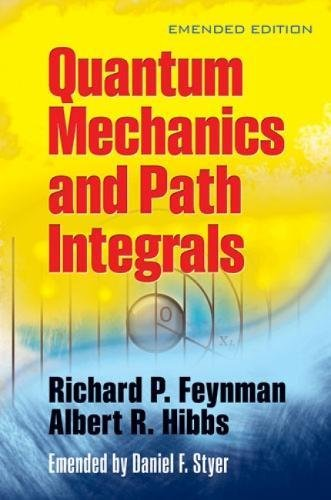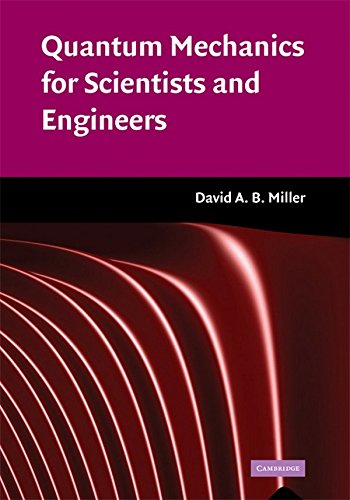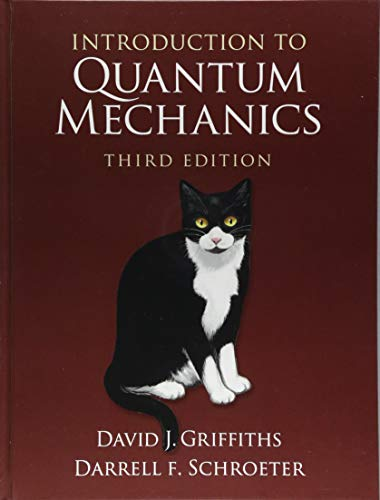# Best Quantum Mechanics Books For Self Study and Research

Quantum Mechanics is the body of scientific laws that describe the wacky behavior of photons, electrons and the other particles that make up the universe. In classical mechanics, objects exist in a specific place at a specific time. However, in quantum mechanics, objects instead exist in a haze of probability; they have a certain chance of being at point A, another chance of being at point B and so on. By reading these best books of Quantum Mechanics you can learn all about the theories and mathematics of Quantum Mechanics with real-life examples.

Here you will get the some of the best Quantum Mechanics Books

## 1. Principles of Quantum Mechanics, 2nd Edition

Principles of Quantum Mechanics is the second edition of one of the most popular Quantum Mechanics book written by famous author R. Shankar. This new edition is revised and comes with plenty of new ideas and concepts. The author first introduces a reader with the prerequisites needed to learn before learning Quantum Mechanics. Then he takes you to a journey from classical physics to Quantum Physics. He does the discussion of complex topics chapter wise which will help a reader to understand Quantum Mechanics. Also in this book, there are entirely re-written mathematics, a discussion of Time-reversal invariance, and extensive coverage of a variety of path integrals and their applications. All that comes with plenty of examples.

Key topics:

• Mathematical Introduction and review of Classical Mechanics
• A review of Newtonian, Lagrangian, and Hamiltonian mechanics
• One dimension problems
• The Harmonic Oscillator
• Path Integral Formulation of Quantum Theory
• The Heisenberg Uncertainty Theorem
• Rotational in-variance and Angular Momentum
• Time-dependent and independent Perturbation Theory
• The Dirac Equation, Path Integrals and many more

## 2. Lectures on Quantum Mechanics

Lectures on Quantum Mechanics: 2nd Edition is written by one of the greatest living Physicist and a Noble Laureate in Physics STEVEN WEINBERG. This is by far the finest course in quantum mechanics you will find. It contains the whole formalism, fully explained, and wholly up to date. In this book, Weinberg explains the complex topics of Quantum Mechanics in a very easy and detailed way. It is ideally suited for a graduate course and also a useful reference for researchers. There are some topics in this book which you will not usually find in others. Some highlights: alternatives to the Copenhagen interpretation, Bloch waves and band structure, the Wigner-Eckart theorem, magic numbers, isospin symmetry, the Dirac theory of constrained canonical systems, general scattering theory, the optical theorem and many more. This is a must-have book for all who are learning Quantum Mechanics.

This book includes:

• Historical Introduction to Atoms, Particles, Photons and Quantum Mechanics
• Schrodinger Equation
• General Principles of Quantum Mechanics
• Spin
• Approximation for energy Eigenvalues
• Time-dependent problems
• Potential Scattering
• The Canonical Formalism
• The Quantum Theory of radiation
• Entanglement

## 3. Quantum Mechanics and Path Integrals: Emended Edition (Dover Books on Physics)Author: Richard P. Feynman,Albert R. Hibbs
Published at: 21/07/2010
ISBN: 0486477223

Quantum Mechanics and Path Integrals presents the formulation of quantum mechanics is a description of quantum theory that generalizes the action principle of classical mechanics. Nobel Prize-winning physicist Richard P. Feynman, presents unique insights into this method and its applications of Path Integral in Quantum Mechanics. His presentation style will amaze you as he avoids the dense discussion and presents the theory in a clear, concise and easily understandable way maintaining the balance between mathematics and physics. The first chapters explore the fundamental concepts of quantum mechanics and introduce you with path integrals. The later chapters of this Quantum Mechanics book cover more advanced topics, including the perturbation method, quantum electrodynamics, and the relation of path integrals to statistical mechanics.

Chapters include:

• Fundamental concepts of Quantum Mechanics
• Law of motion
• Schrodinger's description of Quantum Mechanics
• Measurements and operators
• The perturbation method in Quantum Mechanics
• Harmonic Oscillators
• Quantum Electrodynamics
• Statistical Mechanics and many more

## 4. Quantum Mechanics for Scientists and EngineersAuthor: David A. B. Miller
Published at: 21/04/2008
ISBN: 0521897831

Quantum Mechanics For Scientists and Engineers covers the core principles of Quantum Mechanics with the collaboration of engineering, physics, and nanotechnology. This book illustrates theoretical concepts with examples of nano-structured materials, optics, and semiconductor devices. It also covers the basic quantum mechanical scenarios, operators, the uncertainty principle, matrix formalism, Dirac notation, angular momentum, spin, and the Hydrogen atom. In addition, more advanced topics, such as perturbation theory, the density matrix, and approximation techniques are also discussed. David A. B. Miller also relates much of the material to photonics topics, such as absorption, Fermi's Golden Rule, non-linear effects, refractive index, and much more. This book is very useful and can be used as a reference book for both students and engineers.

Topics include:

• Introduction to Quantum Mechanics
• Schrodinger's Equation
• Functions, Operators and Quantum Mechanics
• Perturbation Theory
• Angular momentum
• Methods for the one-dimensional problem
• Spin
• Fermion Operators
• Worked examples and more than 160 homework problems

## 5. Introduction to Quantum MechanicsAuthor: David J. Griffiths,Darrell F. Schroeter
Published at: 16/08/2018
ISBN: 1107189632

Introduction To Quantum Mechanics provides a deep and clear understanding of the complex topics of Quantum Mechanics. David J. Griffiths straightforward approach to the essentials topics are easy to understand and follow. It covers topics like wave equation, Schrodinger's Equation, Angular and Radial Equation, Atoms, helium and solids, space dimension, parity in 1D/3D and different kinds of application of Quantum Mechanics.

Topics include:

• Time-independent Schrodinger's Equation
• Hilbert space
• The uncertainty principle
• 3D quantum mechanics
• Spin
• Two particle system
• Symmetries and conservation law
• Perturbation Theory
• The variational principle
• Scattering
• Adiabatic Theorem and many more

## 6. Quantum Mechanics: Basic & Advanced Concepts for Beginners

This book covers basic to advanced concepts of Quantum Mechanics. This book is detailed with plenty of mathematics which will help you understand the critical concepts. This book explains the meaning and intuition behind theoretical concepts and mathematics. This book is well suited for a beginner who has limited physics and mathematics background. The material covered is broad, from elementary Quantum Mechanics to Quantum Field Theory. The topics beyond Quantum Mechanics have introduced as well including Super-symmetry and String Theory.

Chapters include:

• Classical Mechanics
• Law of Quantum Mechanics
• Hydrogen Atom
• Spin
• Quantum Computing
• Approximation Methods
• Einstein's Special Theory of Relativity
• Quantum Field Theory

Thanks for reading this post. If you have any opinion don't hesitate to comment here. Also please subscribe our newsletter to get more updates.